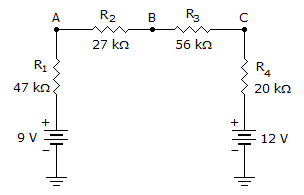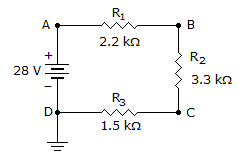# Electronics - Series Circuits

### Exercise :: Series Circuits - General Questions

6.

With a total resistance of 3300 ohms and a 45 V source, if R3 is 1200 ohms, what will be its voltage drop?

 A. 16.36 V B. 32.72 V C. 10.90 V D. 15.00 V

Explanation:

No answer description available for this question. Let us discuss.

7.Calculate the voltage at point B in the given circuit.

 A. +10.48 V B. +0.94 V C. +2.6 V D. +3 V

Explanation:

No answer description available for this question. Let us discuss.

8.In the given circuit, what type of failure will cause the voltage at point B to equal the voltage at point C?

 A. R1 shorts B. R2 shorts C. R3 shorts D. R2 opens

Explanation:

No answer description available for this question. Let us discuss.

9.What is the voltage at points B to D in the given circuit?

 A. +19.2 V B. +8.8 V C. +28 V D. –19.2 V

Explanation:

No answer description available for this question. Let us discuss.

10.Calculate the voltage at point C in the given circuit.

 A. +1.48 V B. +0.94 V C. +11.6 V D. +3 V